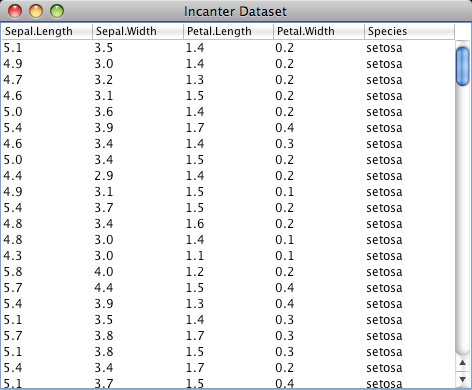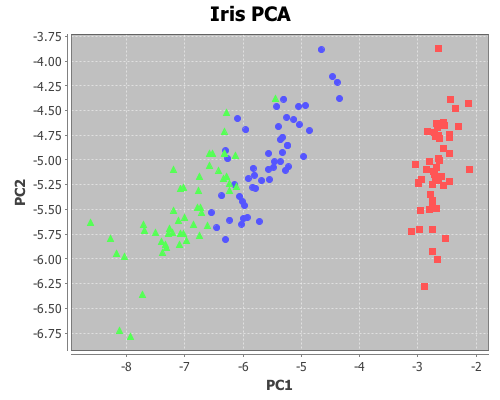# Category Archives: pca

## Principal components analysis

Principal components analysis (PCA) is often used to reduce the number of variables, or dimensions, in a data set in order to simplify analysis or aid in visualization. The following is an example of using it to visualize Fisher’s five-dimensional iris data on a two-dimensional scatter plot, revealing patterns that would be difficult to detect otherwise.

First, principal components will be extracted from the four continuous variables (sepal-width, sepal-length, petal-width, and petal-length); next, these variables will be projected onto the subspace formed by the first two components extracted; and then this two-dimensional data will be shown on a scatter-plot. The fifth dimension (species) will be represented by the color of the points on the scatter-plot.

For this example, you will need the incanter.core, incanter.stats, incanter.charts, and incanter.datasets libraries. The incanter.datasets library contains sample data sets.

``(use '(incanter core stats charts datasets))``

For more information on using these packages see the matrices, datasets, and sample plots pages on the Incanter wiki.

Next, load the iris dataset and view it.

``````(def iris (to-matrix (get-dataset :iris)))
(view iris)``````Then, extract the columns to use in the PCA,

``(def X (sel iris :cols (range 4)))``

and extract the “species” column for identifying the group.

``(def species (sel iris :cols 4))``

Run the PCA on the first four columns only

``(def pca (principal-components X))``

Extract the first two principal components

``````(def components (:rotation pca))
(def pc1 (sel components :cols 0))
(def pc2 (sel components :cols 1))``````

Project the four dimension of the iris data onto the first two principal components

``````(def x1 (mmult X pc1))
(def x2 (mmult X pc2))``````

Now plot the transformed data, coloring each species a different color

``````(view (scatter-plot x1 x2
:group-by species
:x-label "PC1"
:y-label "PC2"
:title "Iris PCA"))``````The complete code for this example can be found here.